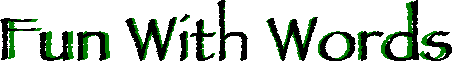Main      Site Guide### Numbers

There are a number of interesting observations that can be made about numbers, as spelled out in English. Here are a few:

### Miscellaneous Facts

Eight is the first number alphabetically. Zero is the last.

Four is the only number that, spelled out, has as many letters.

Fifty and seventy are the only numbers divisible by ten that, when spelled out, have as many letters as ten divides into them. Therefore, fifty-four and seventy-four are equal to the numbers you get when you count the letters digit by digit. Thirty-six and forty-five have this same property in reverse, that is, the first word has the same number of letters as the second digit, and vice versa.

Forty is the only number whose letters are in alphabetical order. One is the only number whose letters are in reverse alphabetical order. First also has its letters in alphabetical order.

Eighty-eight, eleven letters long, is the longest number that is normally typed using strictly alternating hands (ignoring the hyphen).

Interchangeability is the word in the English language that contains the letters to form the most numbers. Its letters can form the words three, eight, nine, ten, thirteen, thirty, thirty-nine, eighty, eighty-nine, ninety, and ninety-eight.

Lewis Carroll, the author of Alice In Wonderland, once noted that "eleven plus two" and "twelve plus one" use the same letters and produce the same sum.

### First Appearances of Letters

• One thousand is the first number that, when spelled out, contains an a. (One hundred and one if you count the "and.")
• One billion is the first number that contains a b.
• One octillion is the first number that contains a c.
• One hundred is the first number that contains a d.
• Zero is the first number that contains an e. Starting from one, it's one.
• Four is the first number that contains an f.
• Eight is the first number that contains a g.
• Three is the first number that contains an h.
• Five is the first number that contains an i.
• The letters j and k do not appear in the English spelling of any cardinal number.
• Eleven is the first number that contains an l.
• One million is the first number that contains an m.
• One is the first number that contains an n.
• Zero is the first number that contains an o. Starting from one, it's one.
• One septillion is the first number that contains a p.
• One quadrillion is the first number that contains a q.
• Zero is the first number that contains an r. Starting from one, it's three.
• Six is the first number that contains an s.
• Two is the first number that contains a t.
• Four is the first number that contains a u.
• Five is the first number that contains a v.
• Two is the first number that contains a w.
• Six is the first number that contains an x.
• Twenty is the first number that contains a y.
• Zero is the first and only number that contains a z. (One zillion is not a real number.)

Seven is the first number not to contain any letter for the first time.

One octillion, the first number containing a c, is the largest number to contain any letter for the first time.